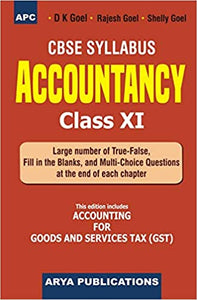# APC'S Accountancy Class- XI

Regular price
Rs. 663.00
Sale price
Rs. 663.00
Regular price
Rs. 680.00
Sold out
Unit price
per

Some of the special features of the book are :

• The book has been written in a simple language keeping in view the standard of Class XI students. This book will also be of great use to those students who are just beginning to learn the subject of Accountancy.

• A number of illustrations have been given in each chapter and these have been solved in such a simple manner that students can easily understand them.

• Practical questions given at the end of each chapter are strictly in the serial order of the illustrations. Answers and hints to solve the questions have been given at the end of each question. We are very much confident that after doing the illustrations, students can themselves solve the practical questions.

• The book contains essay-type, short-answer type, objective type and practical questions at the end of each chapter so that students may test their understanding of the chapter.